# Sound Waves Questions and Answers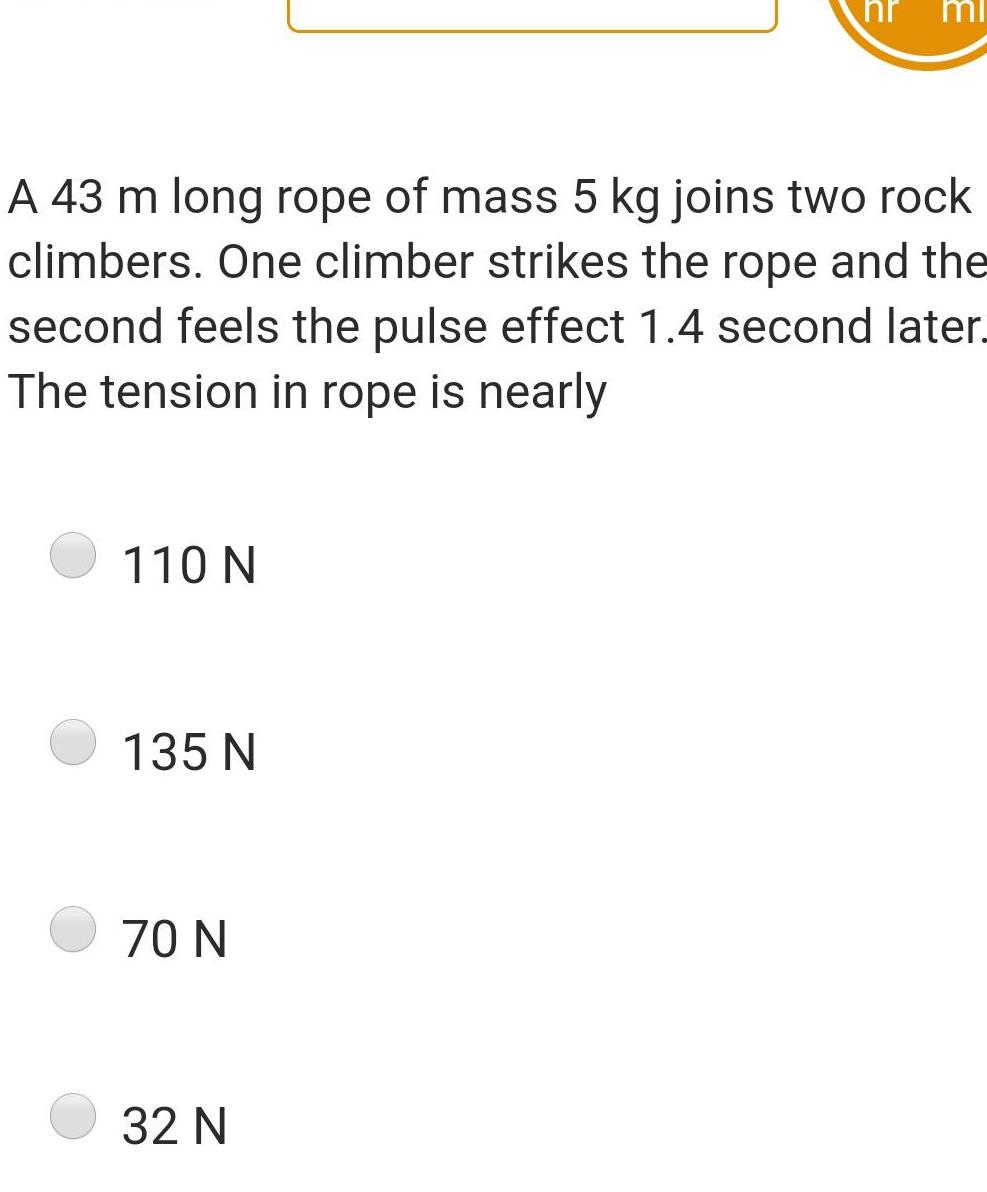Physics
Sound Waves
110 N A 43 m long rope of mass 5 kg joins two rock climbers One climber strikes the rope and the second feels the pulse effect 1 4 second later The tension in rope is nearly 135 N 70 N hr 32 N mi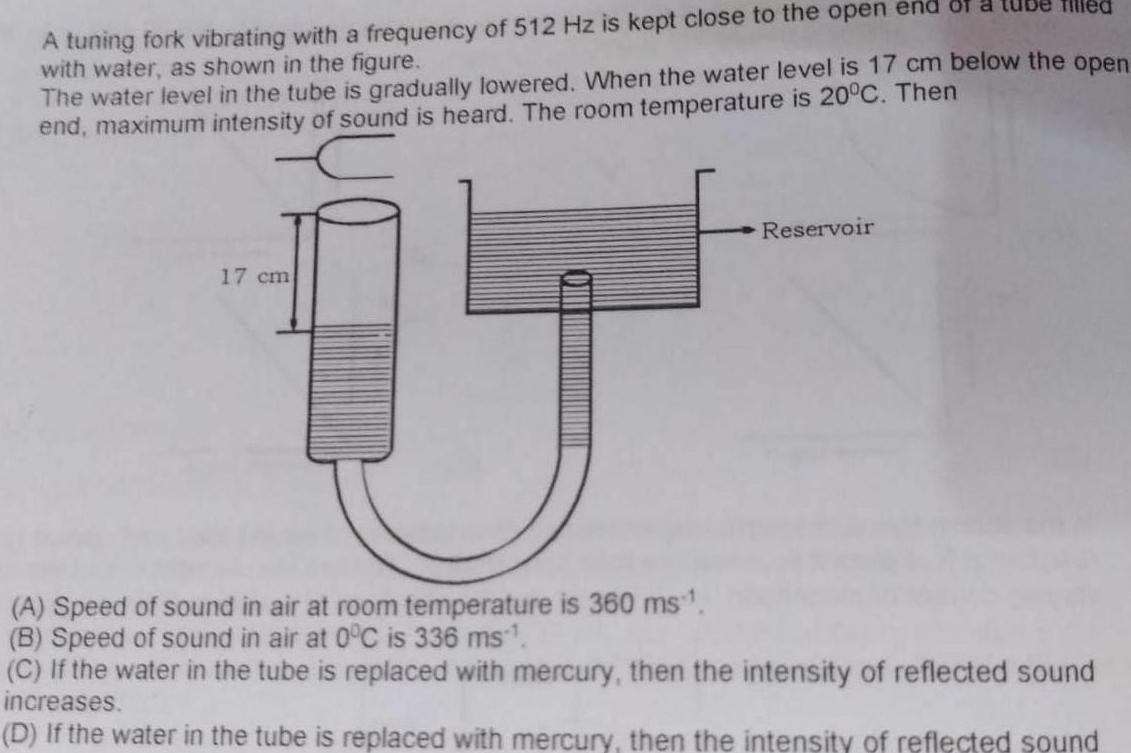Physics
Sound Waves
A tuning fork vibrating with a frequency of 512 Hz is kept close to the open end of a with water as shown in the figure The water level in the tube is gradually lowered When the water level is 17 cm below the open end maximum intensity of sound is heard The room temperature is 20 C Then 17 cm A Speed of sound in air at room temperature is 360 ms B Speed of sound in air at 0 C is 336 ms Tilled Reservoir C If the water in the tube is replaced with mercury then the intensity of reflected sound increases D If the water in the tube is replaced with mercury then the intensity of reflected sound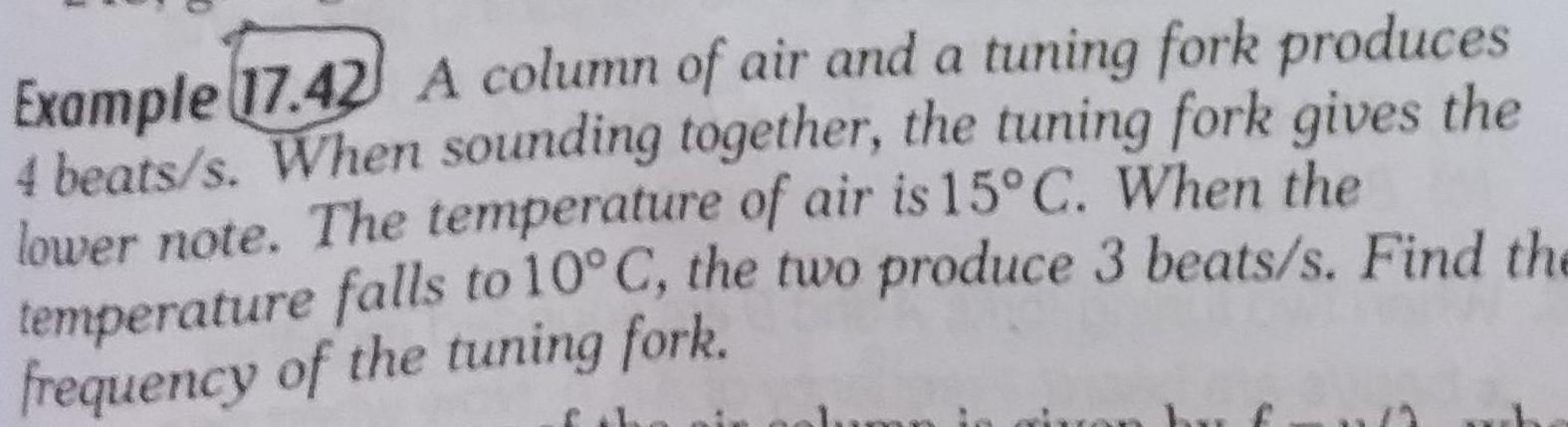Physics
Sound Waves
Example 17 42 A column of air and a tuning fork produces 4 beats s When sounding together the tuning fork gives the lower note The temperature of air is 15 C When the temperature falls to 10 C the two produce 3 beats s Find the frequency of the tuning fork by f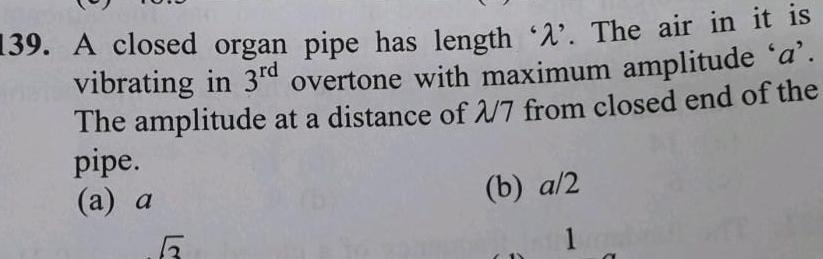Physics
Sound Waves
139 A closed organ pipe has length A The air in it is vibrating in 3rd overtone with maximum amplitude a The amplitude at a distance of 2 7 from closed end of the pipe a a 5 b a 2 1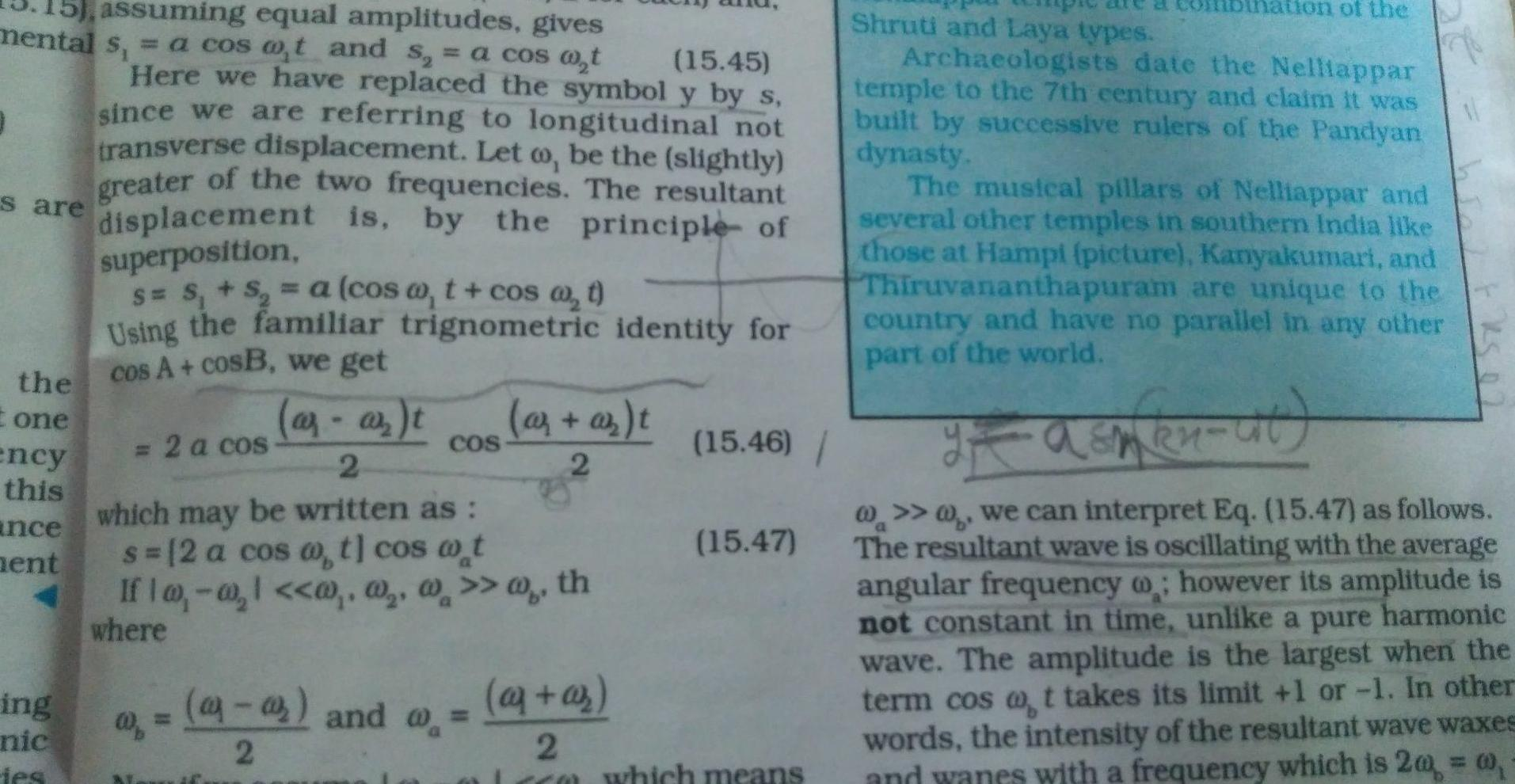Physics
Sound Waves
15 assuming equal amplitudes gives mental s a cos at and s a cos w t 0 s are the one ency this ance ent ing nic ies 15 45 Here we have replaced the symbol y by s since we are referring to longitudinal not transverse displacement Let wo be the slightly greater of the two frequencies The resultant displacement is by the principle of superposition S S S a cosa t cos w t Using the familiar trignometric identity for cos A cosB we get ar ar t 2 which may be written as s 2 a cos w t cos w t 2 a cos COS and 2 az az t 2 If w w 1 w w w w th where 15 46 15 47 aj az 2 cca which means nation of the Shruti and Laya types Archaeologists date the Nelliappar temple to the 7th century and claim it was built by successive rulers of the Pandyan dynasty The musical pillars of Nelliappar and several other temples in southern India like those at Hampi picture Kanyakumari and Thiruvananthapuram are unique to the country and have no parallel in any other part of the world I asmen ut we can interpret Eq 15 47 as follows The resultant wave is oscillating with the average angular frequency however its amplitude is not constant in time unlike a pure harmonic wave The amplitude is the largest when the term cos at takes its limit 1 or 1 In other words the intensity of the resultant wave waxes and wanes with a frequency which is 20 0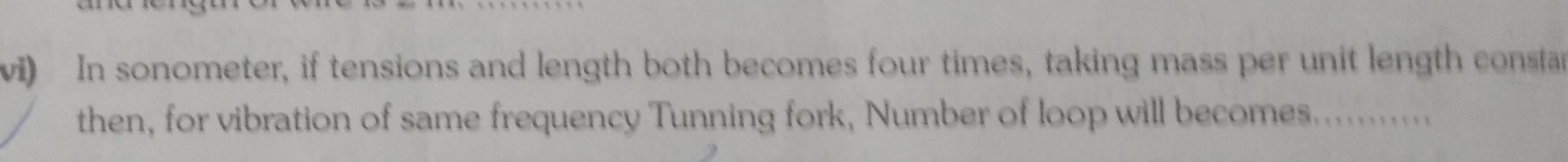Physics
Sound Waves
vi In sonometer if tensions and length both becomes four times taking mass per unit length consta then for vibration of same frequency Tunning fork Number of loop will becomes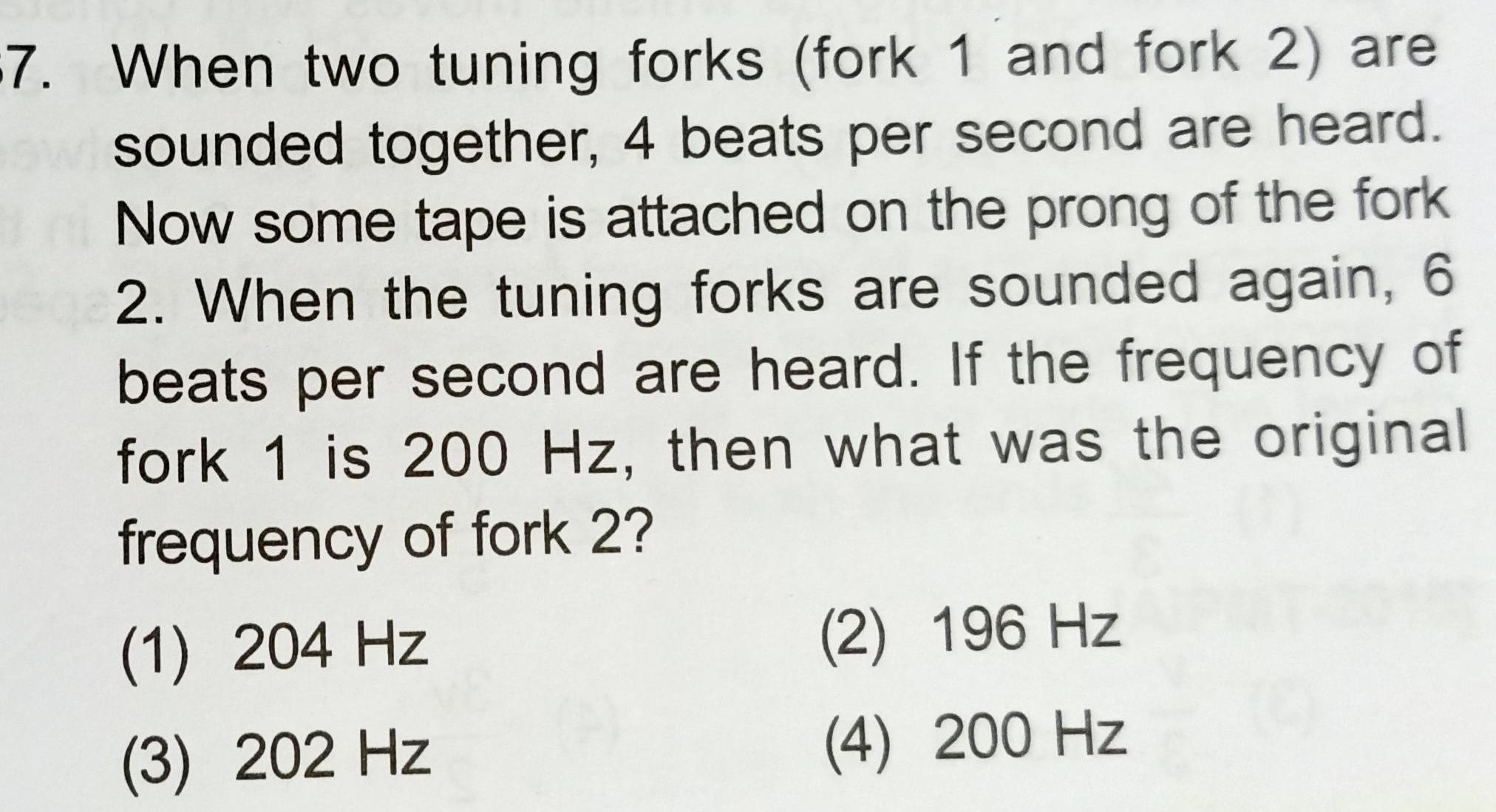Physics
Sound Waves
7 When two tuning forks fork 1 and fork 2 are sounded together 4 beats per second are heard Now some tape is attached on the prong of the fork 602 When the tuning forks are sounded again 6 beats per second are heard If the frequency of fork 1 is 200 Hz then what was the original frequency of fork 2 1 204 Hz 3 202 Hz 2 196 Hz 4 200 Hz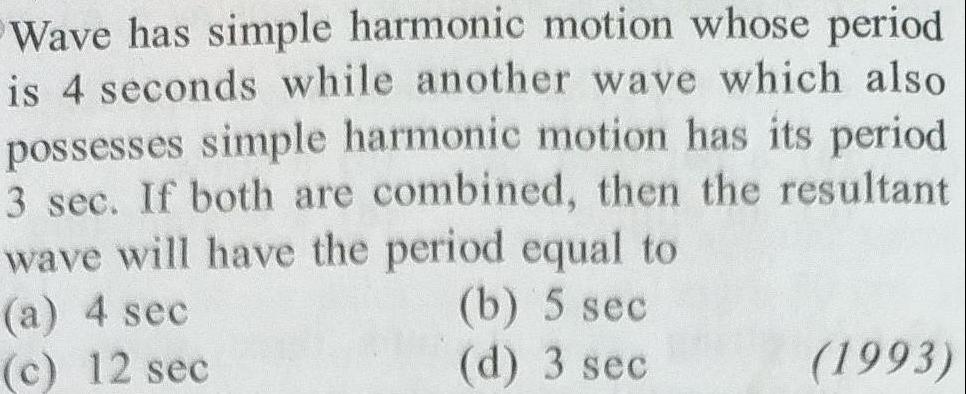Physics
Sound Waves
Wave has simple harmonic motion whose period is 4 seconds while another wave which also possesses simple harmonic motion has its period 3 sec If both are combined then the resultant wave will have the period equal to a 4 sec c 12 sec b 5 sec d 3 sec 1993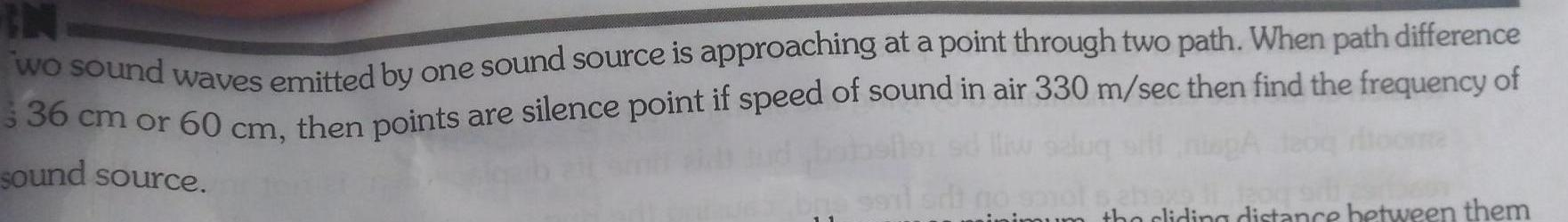Physics
Sound Waves
wo sound waves emitted by one sound source is approaching at a point through two path When path difference 3 36 cm or 60 cm then points are silence point if speed of sound in air 330 m sec then find the frequency of sound source bre hoxs inimum tho cliding distance between them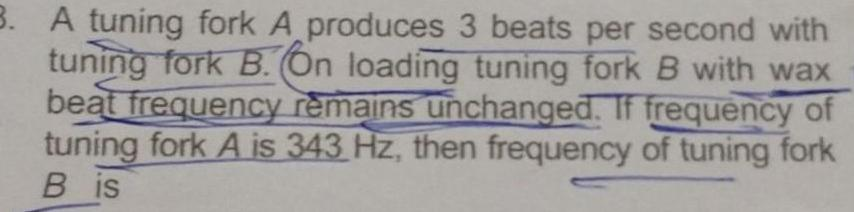Physics
Sound Waves
3 A tuning fork A produces 3 beats per second with tuning fork B On loading tuning fork B with wax beat frequency remains unchanged If frequency of tuning fork A is 343 Hz then frequency of tuning fork Bis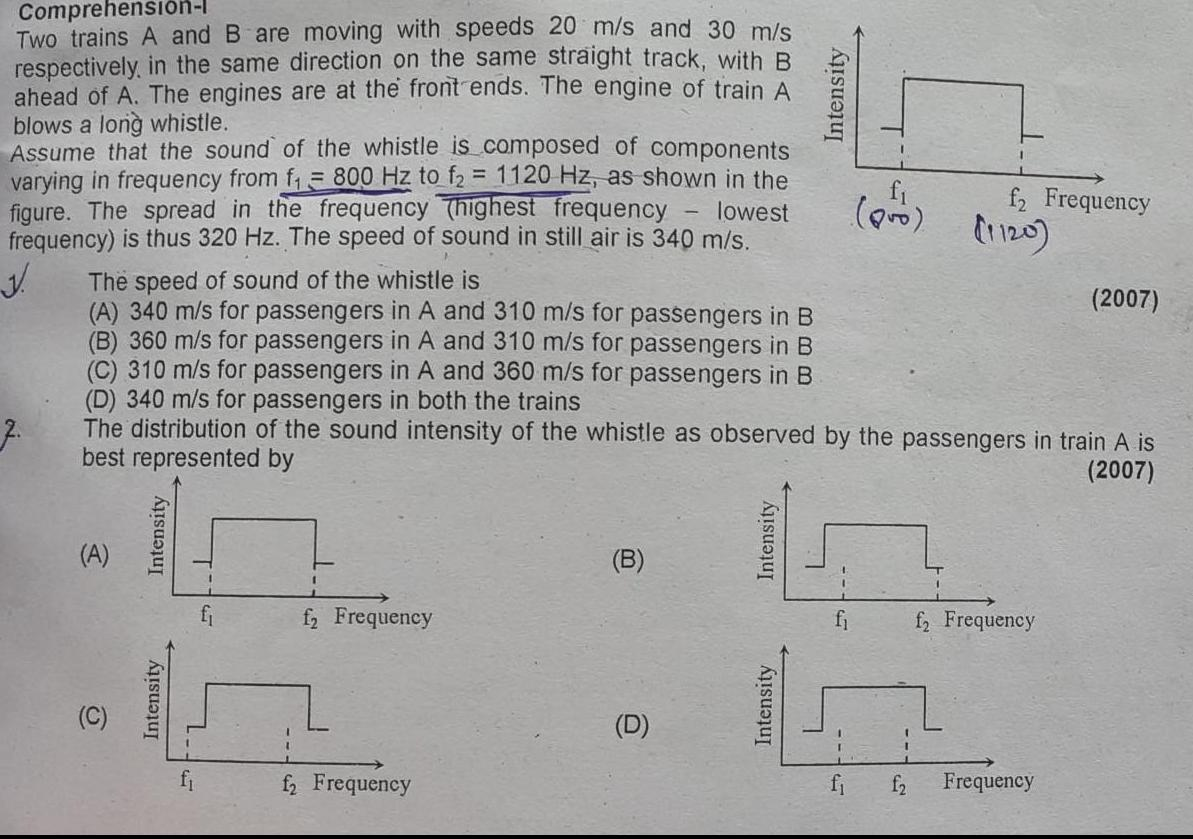Physics
Sound Waves
Comprehension l Two trains A and B are moving with speeds 20 m s and 30 m s respectively in the same direction on the same straight track with B ahead of A The engines are at the front ends The engine of train A blows a long whistle Assume that the sound of the whistle is composed of components varying in frequency from f 800 Hz to f 1120 Hz as shown in the figure The spread in the frequency Thighest frequency lowest frequency is thus 320 Hz The speed of sound in still air is 340 m s J The speed of sound of the whistle is A 340 m s for passengers in A and 310 m s for passengers in B B 360 m s for passengers in A and 310 m s for passengers in B C 310 m s for passengers in A and 360 m s for passengers in B D 340 m s for passengers in both the trains The distribution of the sound intensity of the whistle as observed by the passengers in train A is best represented by 2007 7 A C Intensity Intensity f Frequency f2 Frequency B D Intensity Intensity Intensity f pro f2 Frequency 1120 f Frequency f2 Frequency 2007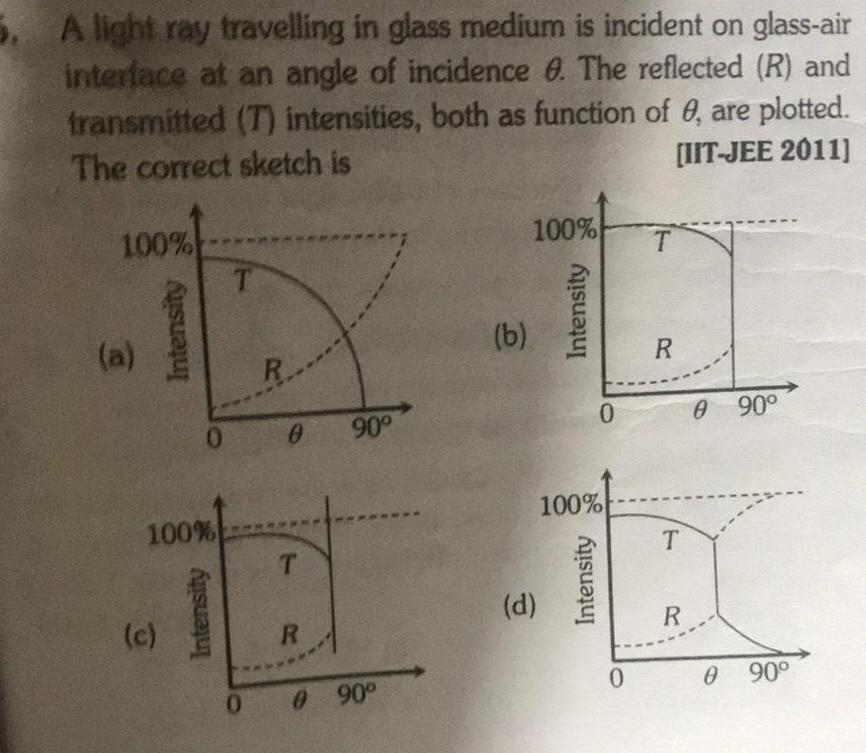Physics
Sound Waves
5 A light ray travelling in glass medium is incident on glass air interface at an angle of incidence 0 The reflected R and transmitted T intensities both as function of 0 are plotted The correct sketch is IIT JEE 2011 100 a Intensity c 0 100 Intensity T R 0 T R 90 0 0 90 b 100 d Intensity 0 100 Intensity 0 T R T R 0 90 0 90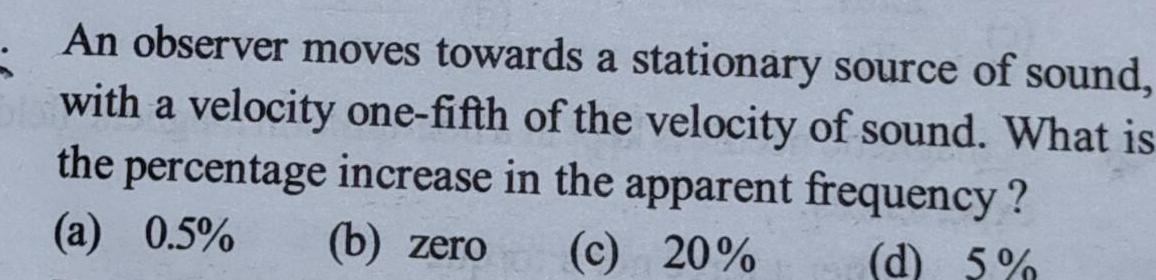Physics
Sound Waves
An observer moves towards a stationary source of sound with a velocity one fifth of the velocity of sound What is the percentage increase in the apparent frequency a 0 5 b zero c 20 d 5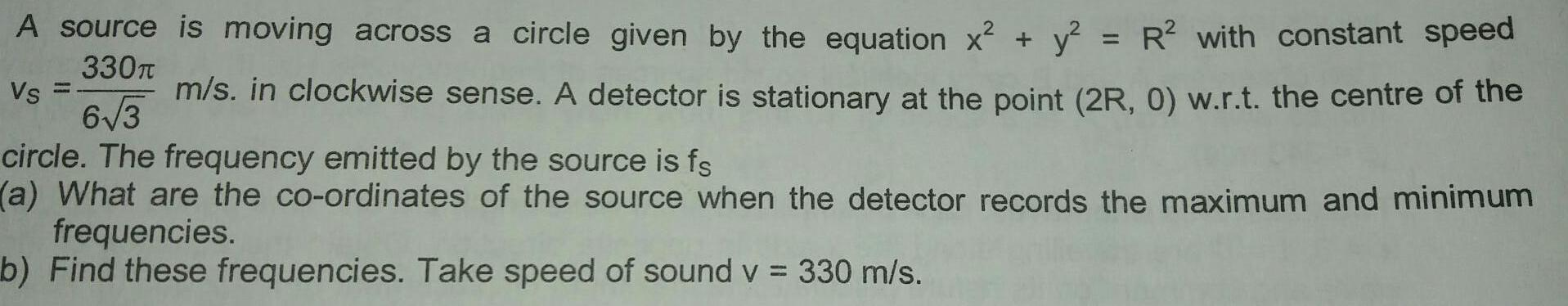Physics
Sound Waves
A source is moving across a circle given by the equation x y R with constant speed m s in clockwise sense A detector is stationary at the point 2R 0 w r t the centre of the 330T Vs 6 3 circle The frequency emitted by the source is fs a What are the co ordinates of the source when the detector records the maximum and minimum frequencies b Find these frequencies Take speed of sound v 330 m s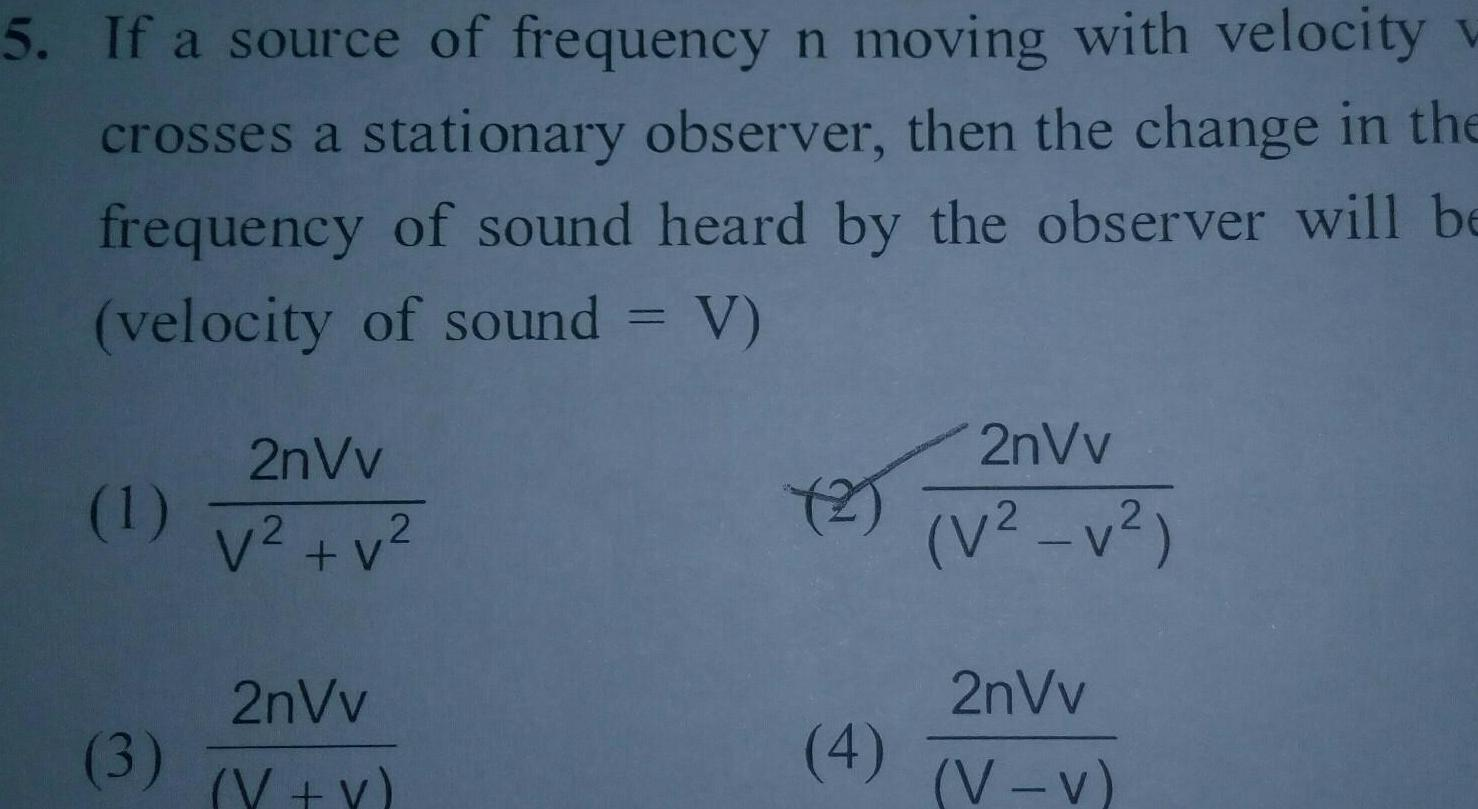Physics
Sound Waves
5 If a source of frequency n moving with velocity crosses a stationary observer then the change in the frequency of sound heard by the observer will be velocity of sound V 1 3 2nVv V v 2nVv V V 2 4 2nVv V v 2nVv V V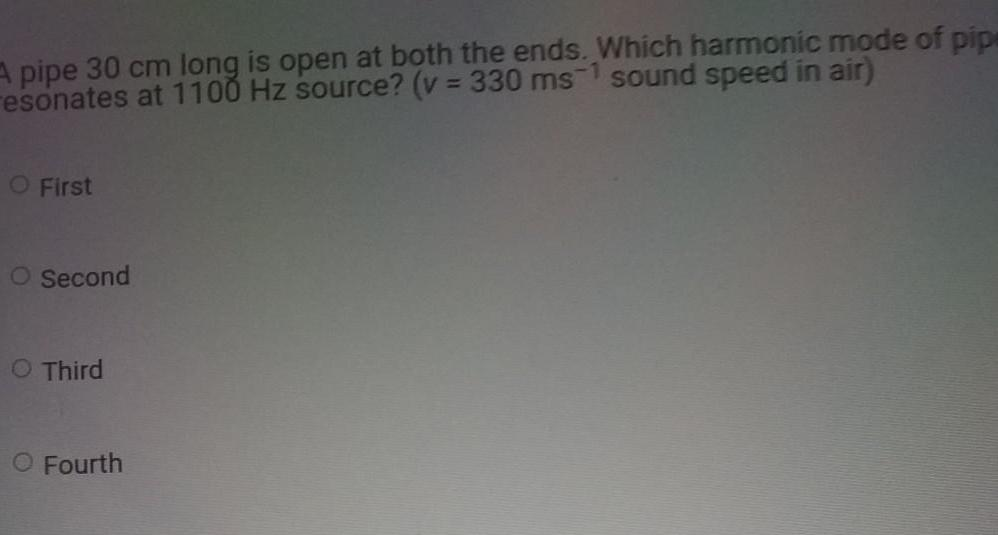Physics
Sound Waves
A pipe 30 cm long is open at both the ends Which harmonic mode of pipe esonates at 1100 Hz source v 330 ms1 sound speed in air O First O Second O Third O Fourth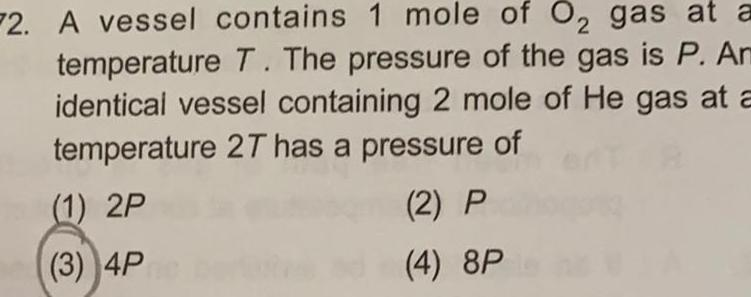Physics
Sound Waves
2 2 A vessel contains 1 mole of O gas at a temperature T The pressure of the gas is P Ar identical vessel containing 2 mole of He gas at a temperature 2T has a pressure of 1 2P 2 P 3 4Pre 4 8P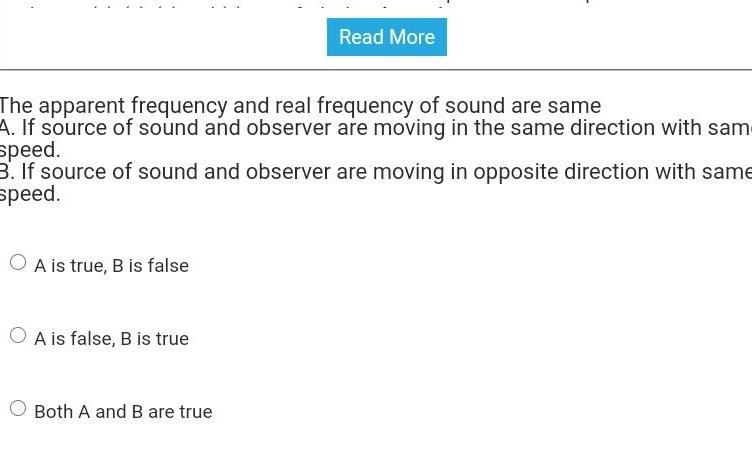Physics
Sound Waves
The apparent frequency and real frequency of sound are same A If source of sound and observer are moving in the same direction with sam speed 3 If source of sound and observer are moving in opposite direction with same speed A is true B is false O A is false B is true Read More Both A and B are true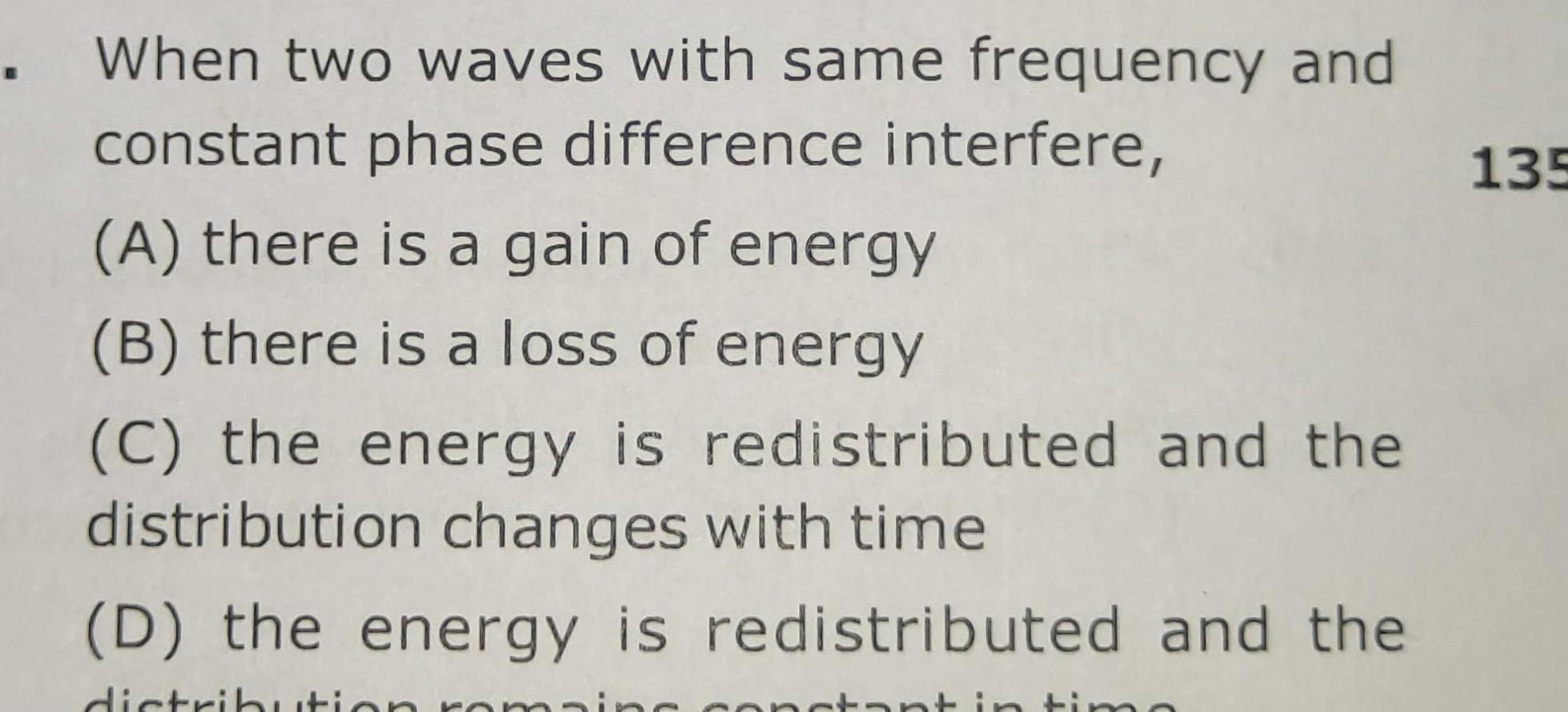Physics
Sound Waves
When two waves with same frequency and constant phase difference interfere A there is a gain of energy B there is a loss of energy C the energy is redistributed and the distribution changes with time D the energy is redistributed and the distribution roma ant in timo 135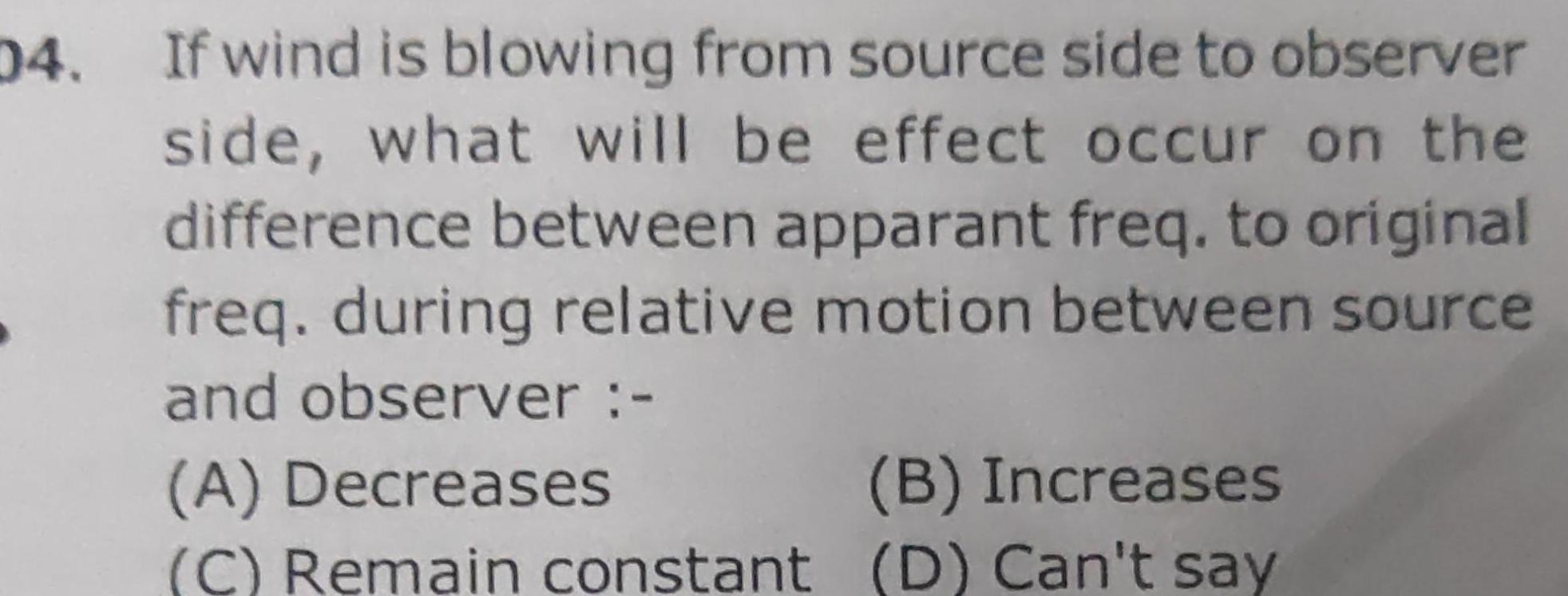Physics
Sound Waves
04 If wind is blowing from source side to observer side what will be effect occur on the difference between apparant freq to original freq during relative motion between source and observer A Decreases B Increases C Remain constant D Can t say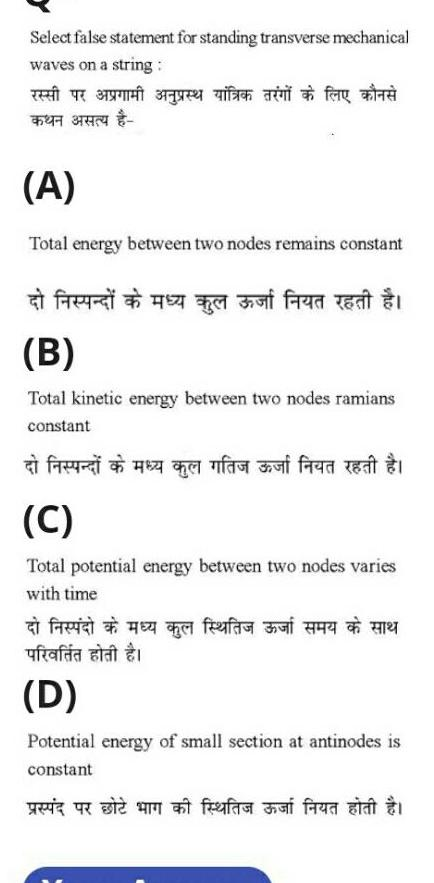Physics
Sound Waves
Select false statement for standing transverse mechanical waves on a string A Total energy between two nodes remains constant fa aft find i B Total kinetic energy between two nodes ramians constant ufafa alat D faut fed r C Total potential energy between two nodes varies with time af Potential energy of small section at antinodes is constant at frafa fara sa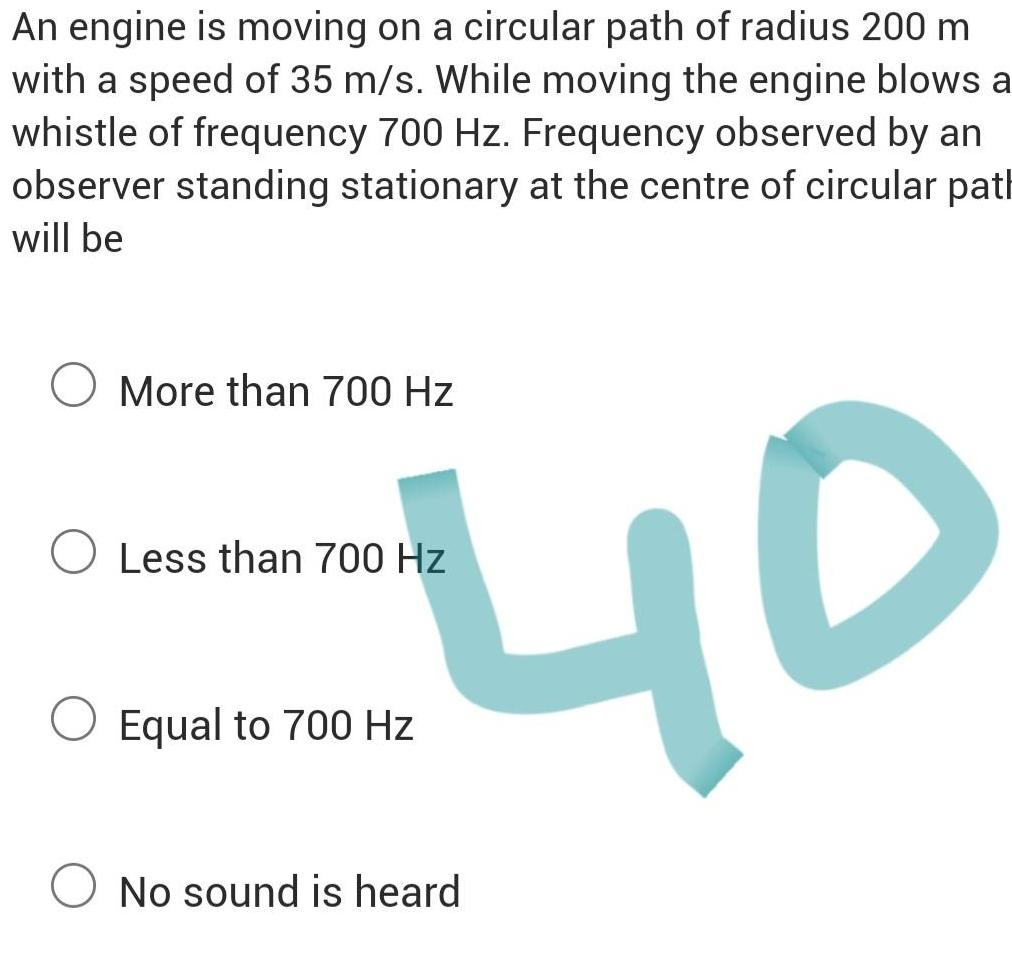Physics
Sound Waves
An engine is moving on a circular path of radius 200 m with a speed of 35 m s While moving the engine blows a whistle of frequency 700 Hz Frequency observed by an observer standing stationary at the centre of circular path will be More than 700 Hz 40 O Less than 700 Hz Equal to 700 Hz O No sound is heard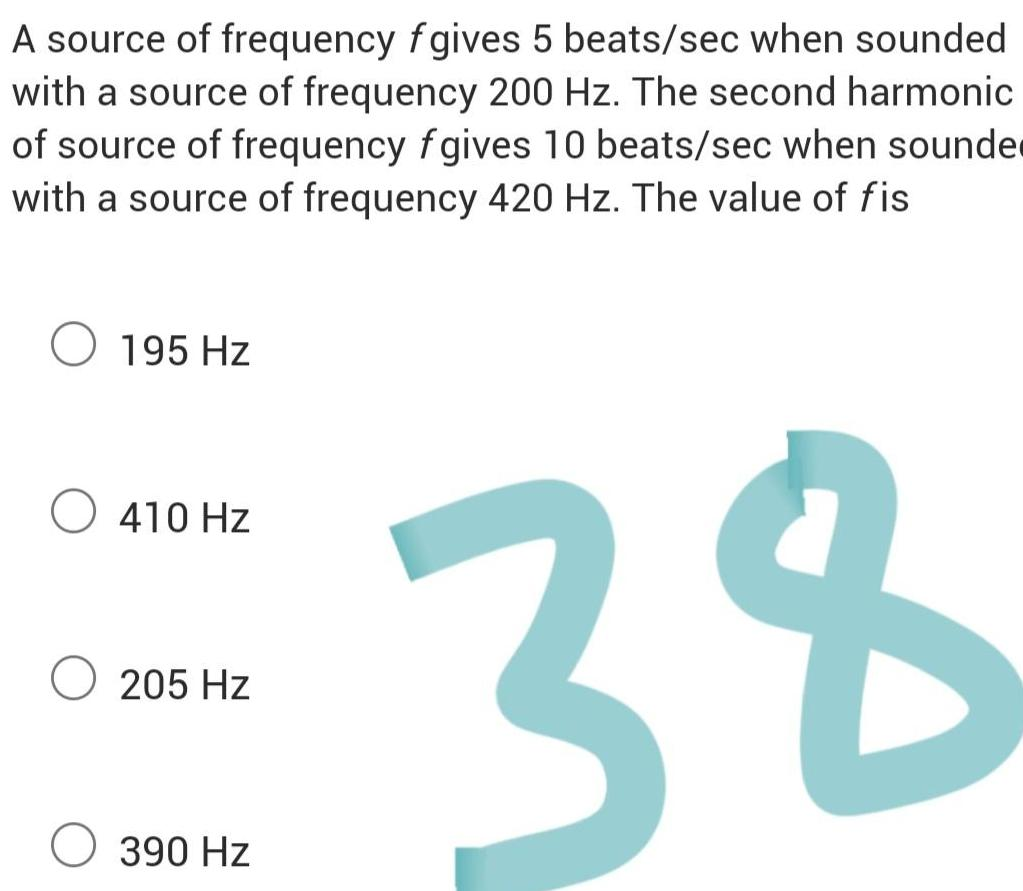Physics
Sound Waves
A source of frequency fgives 5 beats sec when sounded with a source of frequency 200 Hz The second harmonic of source of frequency fgives 10 beats sec when sounde with a source of frequency 420 Hz The value of fis 38 195 Hz O 410 Hz 205 Hz 390 Hz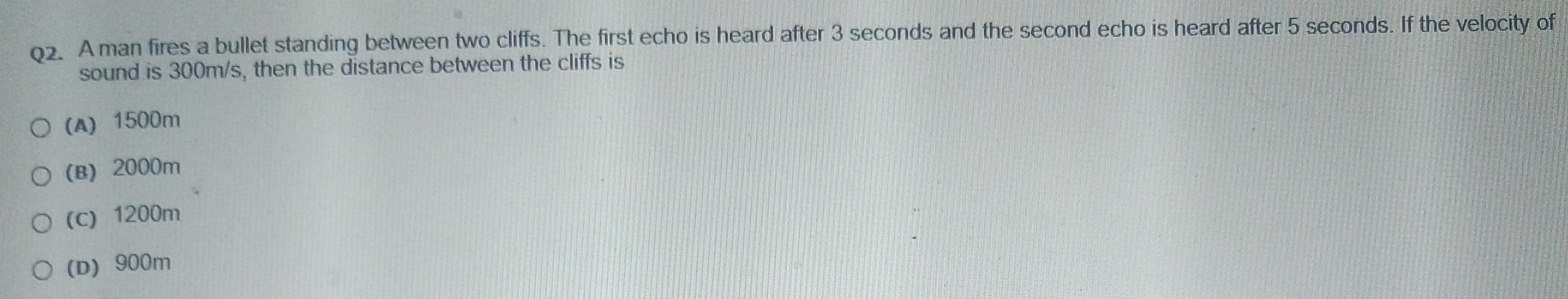Physics
Sound Waves
Q2 A man fires a bullet standing between two cliffs The first echo is heard after 3 seconds and the second echo is heard after 5 seconds If the velocity of sound is 300m s then the distance between the cliffs is O A 1500m O B 2000m O C 1200m O D 900m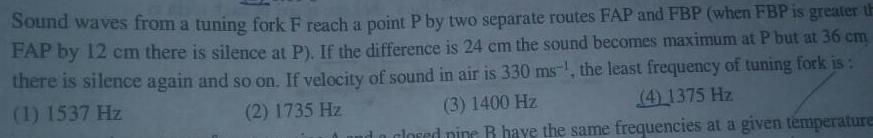Physics
Sound Waves
Sound waves from a tuning fork F reach a point P by two separate routes FAP and FBP when FBP is greater th FAP by 12 cm there is silence at P If the difference is 24 cm the sound becomes maximum at P but at 36 cm there is silence again and so on If velocity of sound in air is 330 ms the least frequency of tuning fork is 1 1537 Hz 2 1735 Hz 3 1400 Hz 4 1375 Hz closed pine R have the same frequencies at a given temperature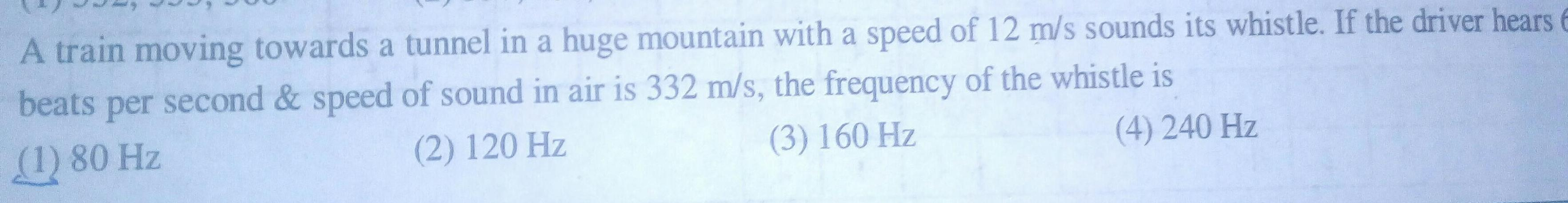Physics
Sound Waves
A train moving towards a tunnel in a huge mountain with a speed of 12 m s sounds its whistle If the driver hears 6 beats per second speed of sound in air is 332 m s the frequency of the whistle is 1 80 Hz 2 120 Hz 3 160 Hz 4 240 Hz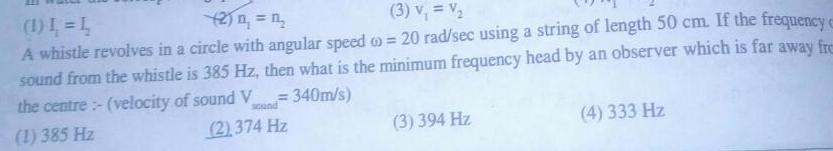Physics
Sound Waves
3 v V 1 L L 2 n A whistle revolves in a circle with angular speed 20 rad sec using a string of length 50 cm If the frequency c sound from the whistle is 385 Hz then what is the minimum frequency head by an observer which is far away fre 340m s the centre velocity of sound V sound 1 385 Hz 2 374 Hz 3 394 Hz 4 333 Hz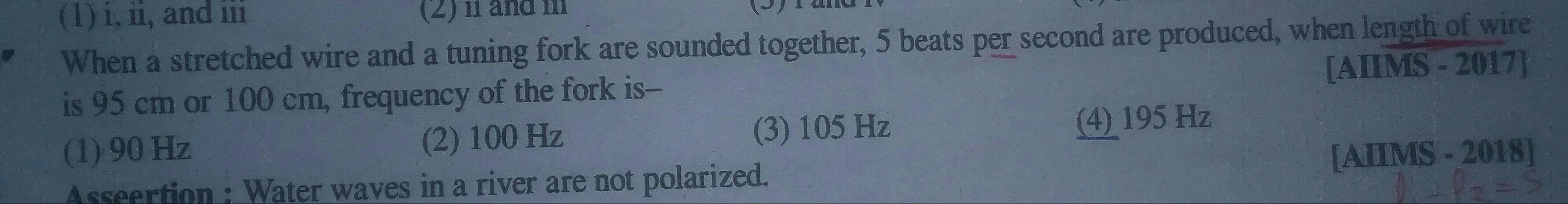Physics
Sound Waves
1 i ii and iii When a stretched wire and a tuning fork are sounded together 5 beats per second are produced when length of wire is 95 cm or 100 cm frequency of the fork is AILMS 2017 1 90 Hz 2 100 Hz 4 195 Hz Asseertion Water waves in a river are not polarized 3 105 Hz AIMS 2018 1 1 5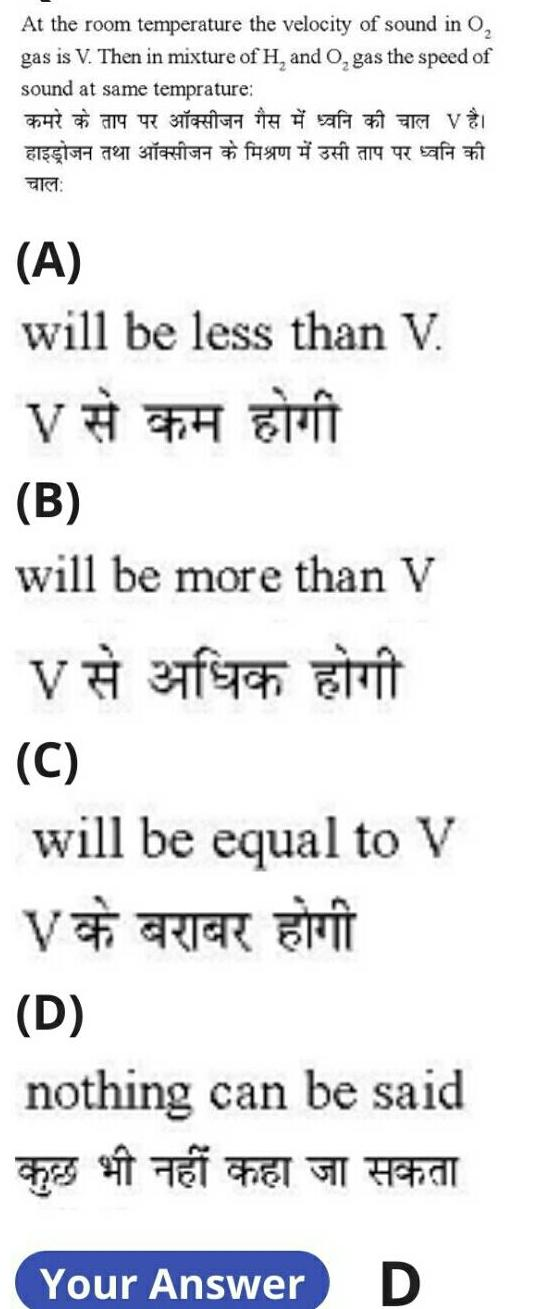Physics
Sound Waves
At the room temperature the velocity of sound in 0 gas is V Then in mixture of H and O gas the speed of sound at same temprature BEGIGH 11 BERIG 49 30 31 F A will be less than V V B will be more than V v 30 t C will be equal to V V CRIER NI D nothing can be said Hal ches Your Answer D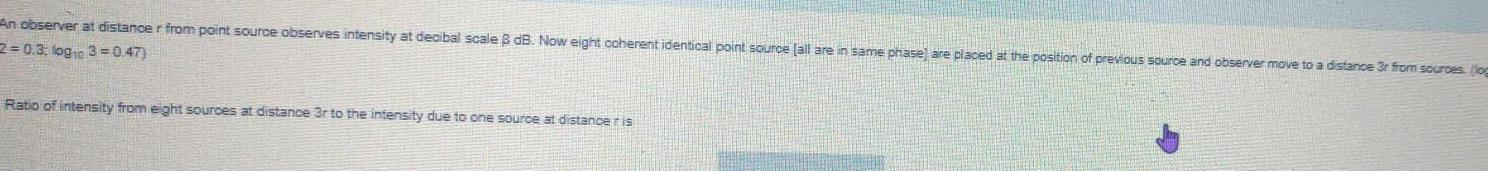Physics
Sound Waves
An observer at distance r from point source observes intensity at decibal scale B dB Now eight coherent identical point source all are in same phase are placed at the position of previous source and observer move to a distance 3r from sources log 2 0 3 logic 3 0 47 Ratio of intensity from eight sources at distance 3r to the intensity due to one source at distance ris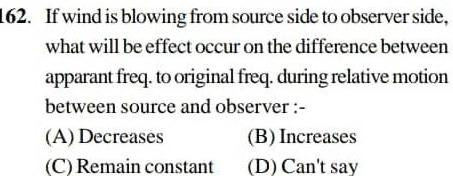Physics
Sound Waves
162 If wind is blowing from source side to observer side what will be effect occur on the difference between apparant freq to original freq during relative motion between source and observer A Decreases B Increases C Remain constant D Can t say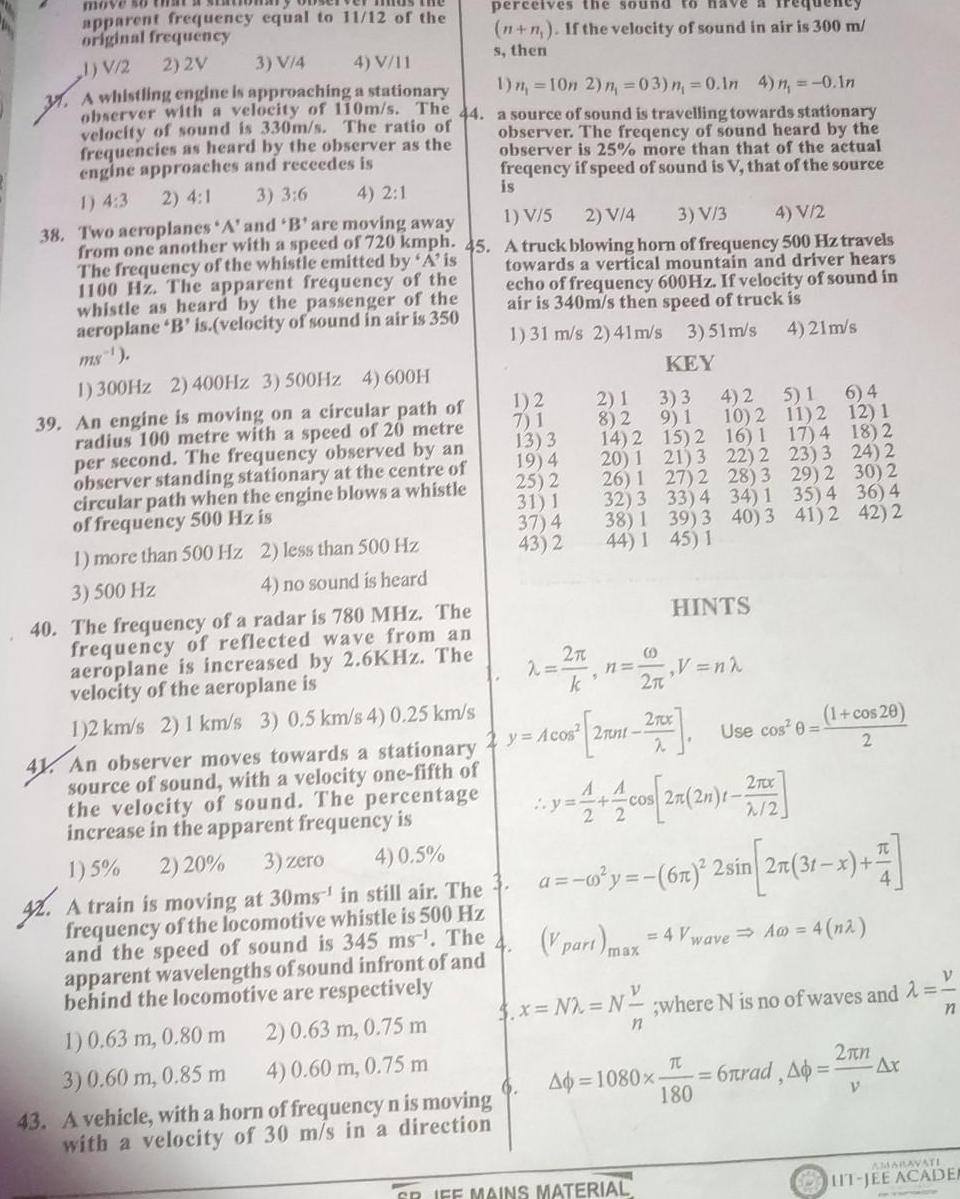Physics
Sound Waves
move apparent frequency equal to 11 12 of the original frequency 1 V 2 2 2V 3 V 4 4 V 11 31 A whistling engine is approaching a stationary y observer with a velocity of 110m s The 44 velocity of sound is 330m s The ratio of frequencies as heard by the observer as the engine approaches and receedes is 1 n 10n 2 n 03 n 0 In 4 n 0 In a source of sound is travelling towards stationary observer The freqency of sound heard by the observer is 25 more than that of the actual freqency if speed of sound is V that of the source 2 4 1 1 4 3 3 3 6 is 4 2 1 1 V 5 2 V 4 3 V 3 4 V 2 38 Two aeroplanes A and B are moving away from one another with a speed of 720 kmph 45 A truck blowing horn of frequency 500 Hz travels The frequency of the whistle emitted by A is 1100 Hz The apparent frequency of the whistle as heard by the passenger of the aeroplane B is velocity of sound in air is 350 ms towards a vertical mountain and driver hears echo of frequency 600Hz If velocity of sound in air is 340m s then speed of truck is 1 31 m s 2 41m s 3 51m s 4 21m s KEY 1 300Hz 2 400Hz 3 500Hz 4 600H 39 An engine is moving on a circular path of radius 100 metre with a speed of 20 metre per second The frequency observed by an observer standing stationary at the centre of circular path when the engine blows a whistle of frequency 500 Hz is 1 more than 500 Hz 2 less than 500 Hz 3 500 Hz 4 no sound is heard perceives the sound n n If the velocity of sound in air is 300 m s then 40 The frequency of a radar is 780 MHz The frequency of reflected wave from an aeroplane is increased by 2 6KHz The velocity of the aeroplane is 1 2 km s 2 1 km s 3 0 5 km s 4 0 25 km s 41 An observer moves towards a stationary source of sound with a velocity one fifth of the velocity of sound The percentage increase in the apparent frequency is 1 5 2 20 3 zero 4 0 5 4 A train is moving at 30ms in still air The frequency of the locomotive whistle is 500 Hz and the speed of sound is 345 ms The apparent wavelengths of sound infront of and behind the locomotive are respectively 1 0 63 m 0 80 m 2 0 63 m 0 75 m 3 0 60 m 0 85 m 4 0 60 m 0 75 m 1 2 7 1 13 3 19 4 25 2 31 1 37 4 43 2 43 A vehicle with a horn of frequency n is moving with a velocity of 30 m s in a direction 2 3 2 k 2 1 3 3 4 2 5 1 6 4 8 2 9 1 10 2 11 2 12 1 14 2 15 2 16 1 18 2 22 2 28 3 34 1 40 3 20 1 26 1 32 3 38 1 44 1 y Acos 2ant 22 21 3 27 2 2 V part max GR IFF MAINS MATERIAL 33 4 39 3 45 1 HINTS V n 270x 2 17 4 23 3 29 2 2TX cos 2n 2n 2 2 Ap 1080x 35 4 41 2 Use cos 0 a y 6m 2 sin 2n 31 x 24 2 30 2 36 4 42 2 1 cos 20 2 3 x N2 N where N is no of waves and V n n 4 Vwave A 4 na T 6nrad Ab 180 2 n V Ax AMARAVATI IT JEE ACADEA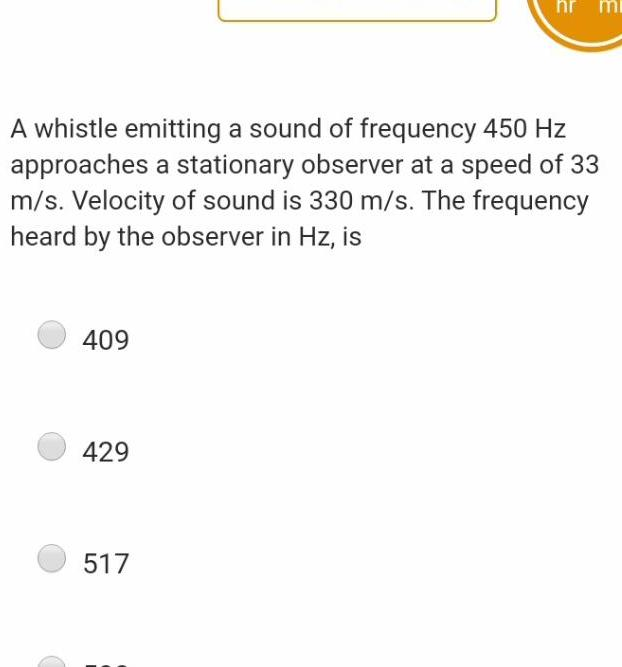Physics
Sound Waves
A whistle emitting a sound of frequency 450 Hz approaches a stationary observer at a speed of 33 m s Velocity of sound is 330 m s The frequency heard by the observer in Hz is 409 429 hr mi 517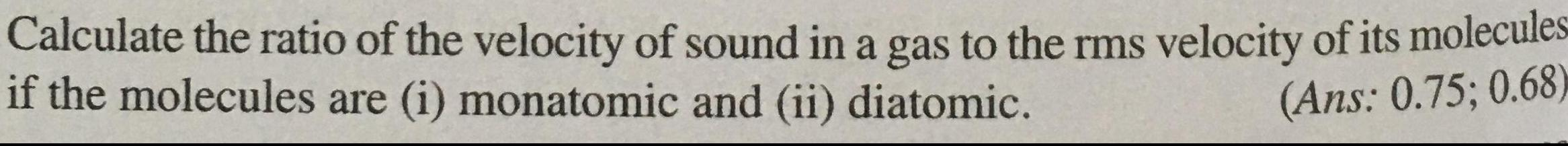Physics
Sound Waves
Calculate the ratio of the velocity of sound in a gas to the rms velocity of its molecules if the molecules are i monatomic and ii diatomic Ans 0 75 0 68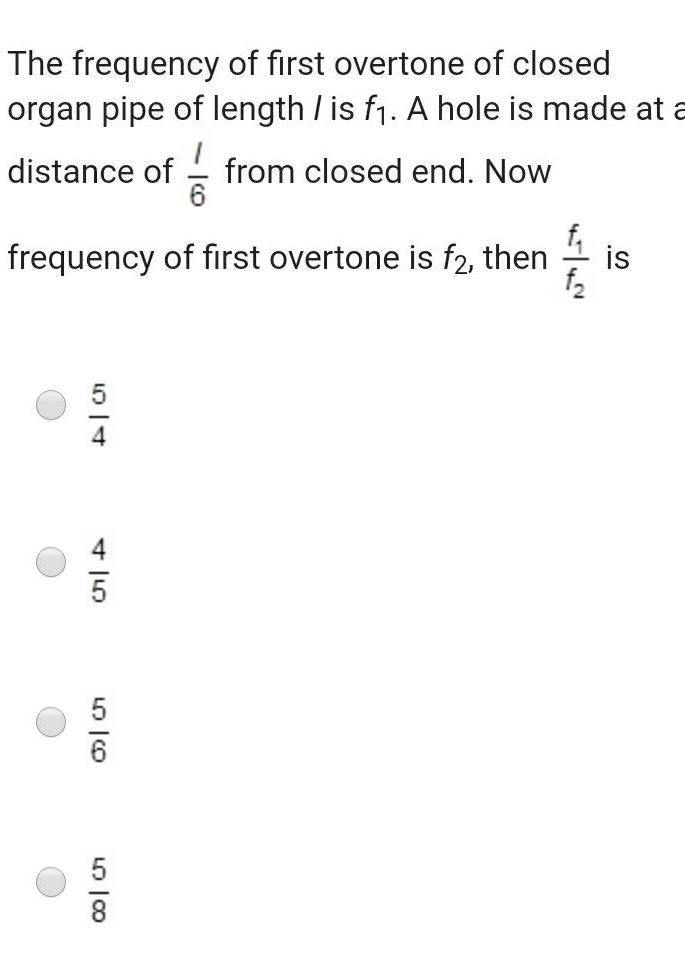Physics
Sound Waves
The frequency of first overtone of closed organ pipe of length is f A hole is made at a 1 distance of from closed end Now 6 frequency of first overtone is f2 then 5 4 56 L 100 is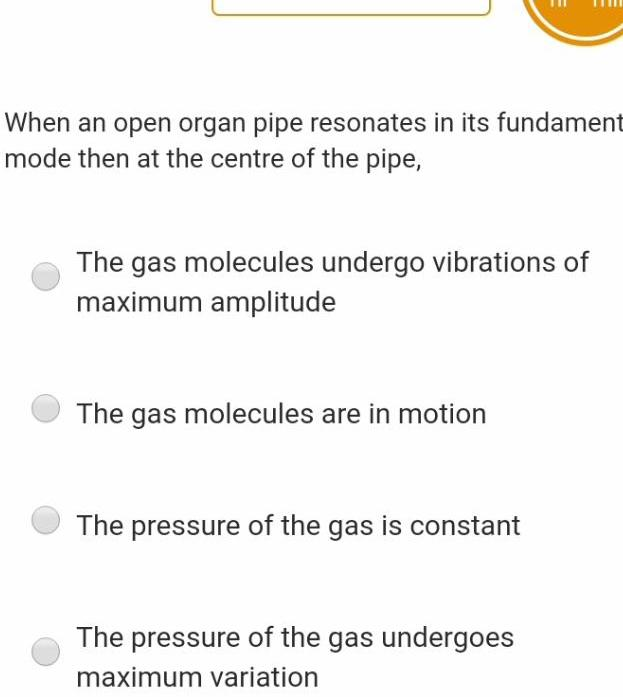Physics
Sound Waves
When an open organ pipe resonates in its fundament mode then at the centre of the pipe The gas molecules undergo vibrations of maximum amplitude The gas molecules are in motion The pressure of the gas is constant The pressure of the gas undergoes maximum variation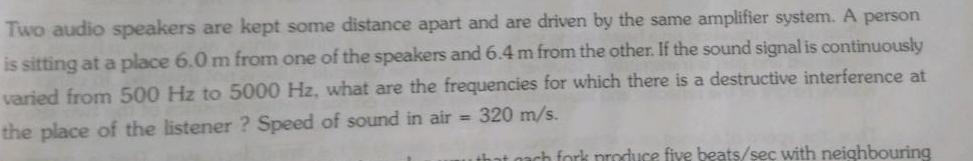Physics
Sound Waves
Two audio speakers are kept some distance apart and are driven by the same amplifier system A person is sitting at a place 6 0 m from one of the speakers and 6 4 m from the other If the sound signal is continuously varied from 500 Hz to 5000 Hz what are the frequencies for which there is a destructive interference at the place of the listener Speed of sound in air 320 m s produce five beats sec with neighbouring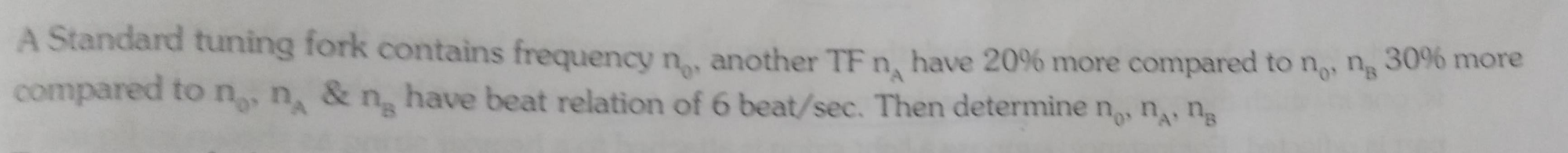Physics
Sound Waves
A Standard tuning fork contains frequency n another TF n have 20 more compared to no na 30 more compared to n n ng have beat relation of 6 beat sec Then determine no n ng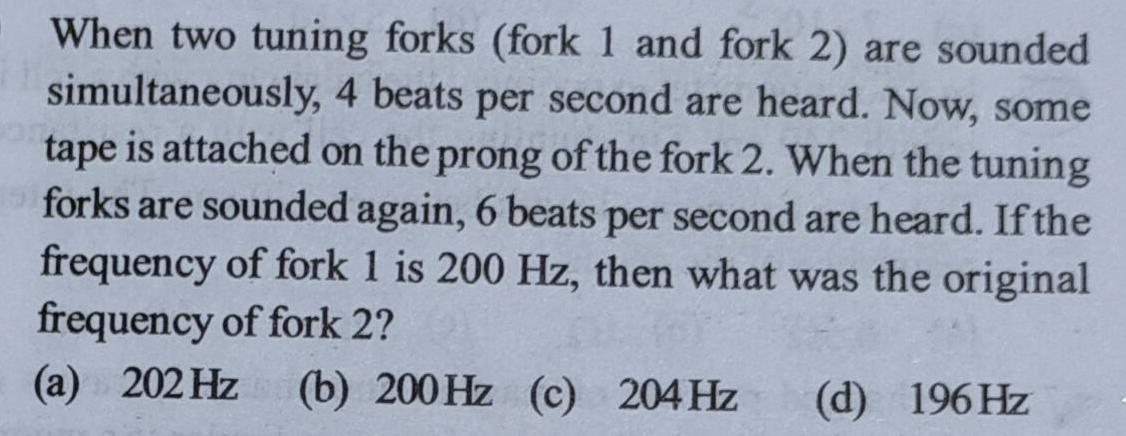Physics
Sound Waves
When two tuning forks fork 1 and fork 2 are sounded simultaneously 4 beats per second are heard Now some tape is attached on the prong of the fork 2 When the tuning forks are sounded again 6 beats per second are heard If the frequency of fork 1 is 200 Hz then what was the original frequency of fork 2 a 202 Hz b 200 Hz c 204 Hz d 196 Hz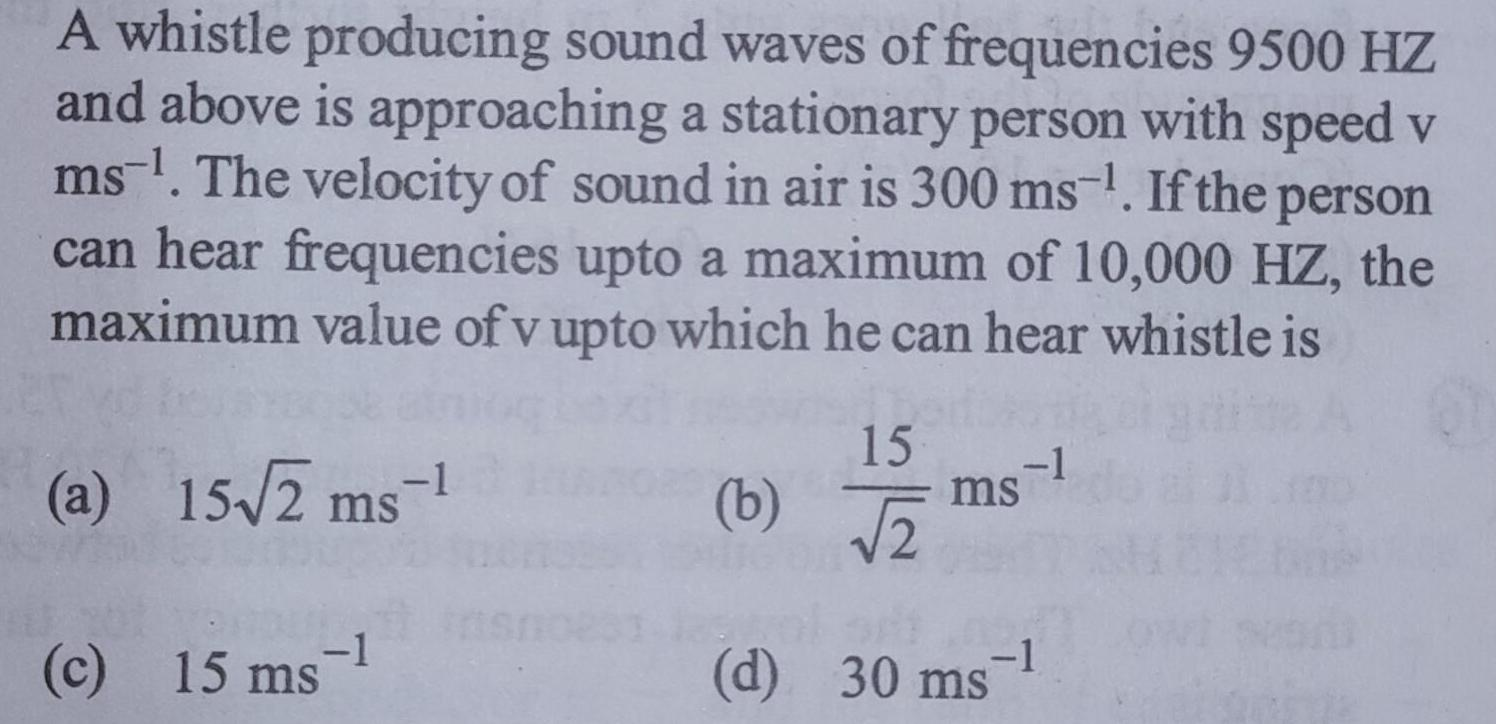Physics
Sound Waves
A whistle producing sound waves of frequencies 9500 HZ and above is approaching a stationary person with speed v ms The velocity of sound in air is 300 ms If the person can hear frequencies upto a maximum of 10 000 HZ the maximum value of v upto which he can hear whistle is a 15 2 ms c 15 ms 1 15 2 b PHIGONV 1 ms d 30 ms 1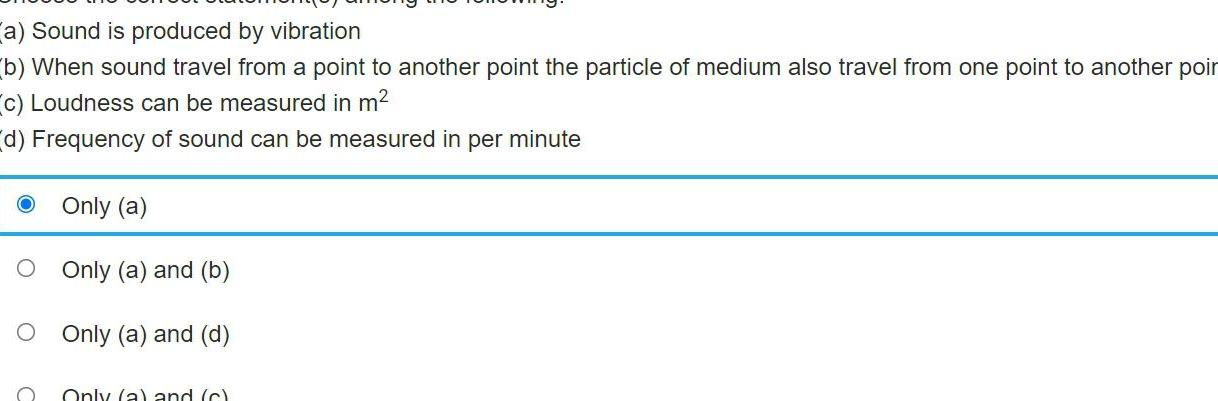Physics
Sound Waves
a Sound is produced by vibration b When sound travel from a point to another point the particle of medium also travel from one point to another poir c Loudness can be measured in m d Frequency of sound can be measured in per minute Only a Only a and b Only a and d Only a and c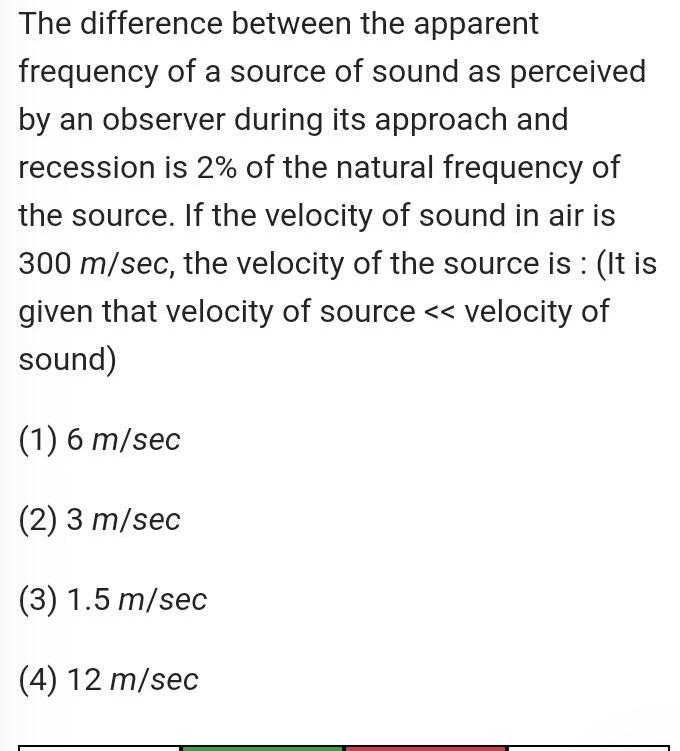Physics
Sound Waves
The difference between the apparent frequency of a source of sound as perceived by an observer during its approach and recession is 2 of the natural frequency of the source If the velocity of sound in air is 300 m sec the velocity of the source is It is given that velocity of source velocity of sound 1 6 m sec 2 3 m sec 3 1 5 m sec 4 12 m sec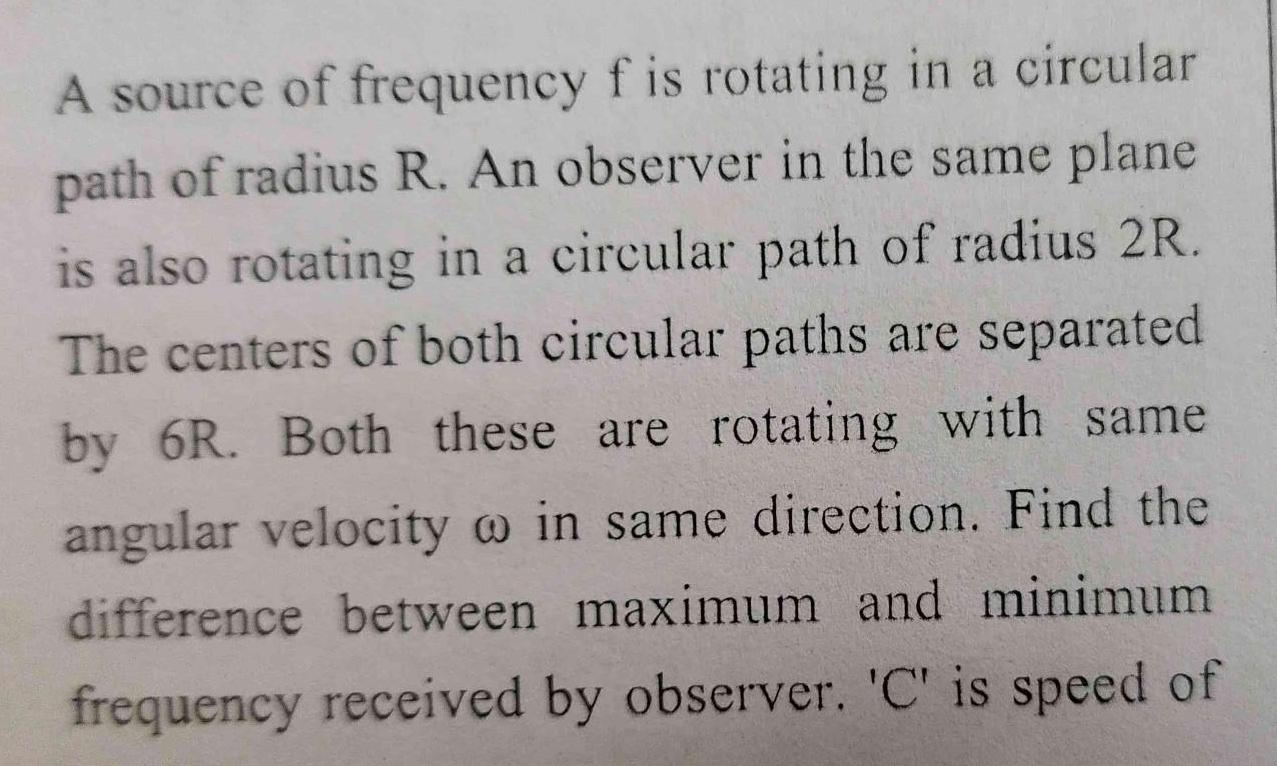Physics
Sound Waves
A source of frequency f is rotating in a circular path of radius R An observer in the same plane is also rotating in a circular path of radius 2R The centers of both circular paths are separated by 6R Both these are rotating with same angular velocity in same direction Find the difference between maximum and minimum frequency received by observer C is speed of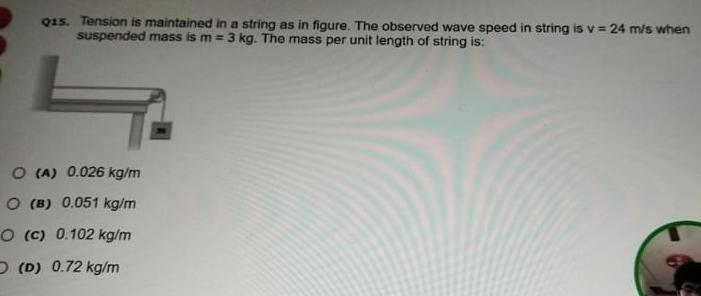Physics
Sound Waves
Q15 Tension is maintained in a string as in figure The observed wave speed in string is v 24 m s when suspended mass is m 3 kg The mass per unit length of string is O A 0 026 kg m O B 0 051 kg m O c 0 102 kg m D D 0 72 kg m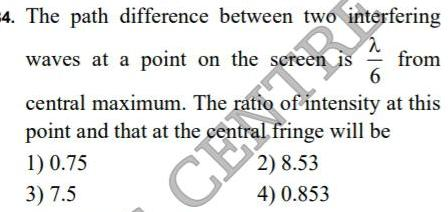Physics
Sound Waves
4 The path difference between two interfering waves at a point on the screen is from 6 central maximum The ratio of intensity at this point and that at the central fringe will be 2 8 53 4 0 853 1 0 75 3 7 5 CENTRA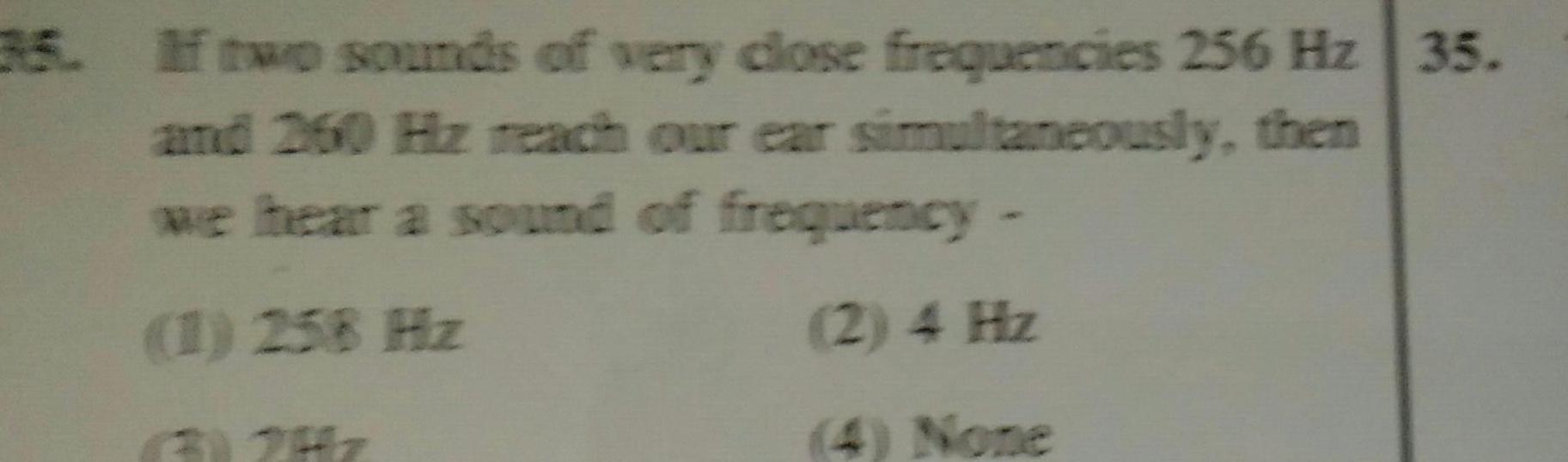Physics
Sound Waves
35 If two sounds of very close frequencies 256 Hz 35 and 260 Hz reach our ear simultaneously then we hear a sound of frequency 1 258 Hz 2 4 Hz 4 None 62Hz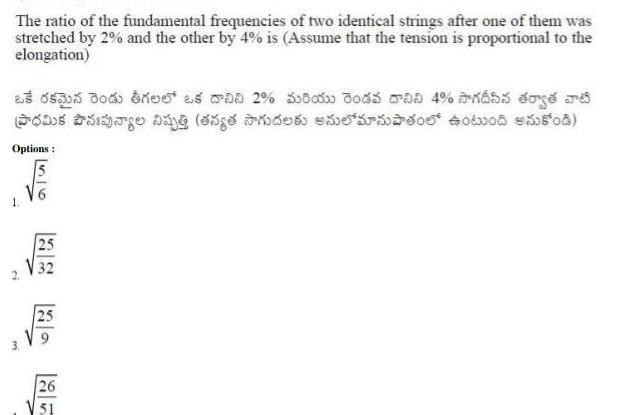Physics
Sound Waves
The ratio of the fundamental frequencies of two identical strings after one of them was stretched by 2 and the other by 4 is Assume that the tension is proportional to the elongation Boned as a 2 58 Jose Day g du Options 25 25 26 51 od 0 4 5 t J r os 40o 508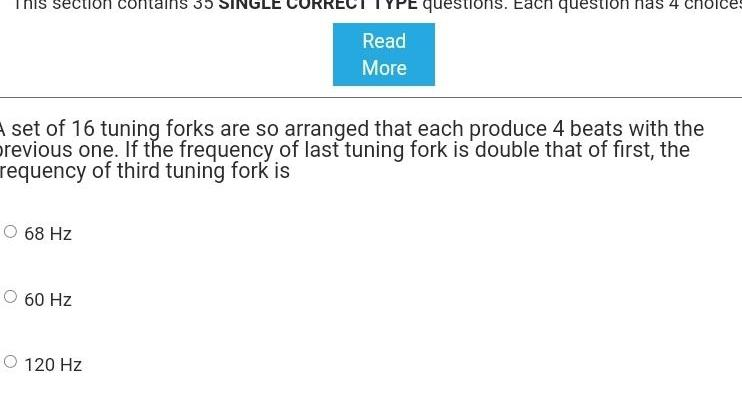Physics
Sound Waves
This section contains 35 O 68 Hz A set of 16 tuning forks are so arranged that each produce 4 beats with the previous one If the frequency of last tuning fork is double that of first the requency of third tuning fork is O 60 Hz Read More O 120 Hz questions Each question has 4 choices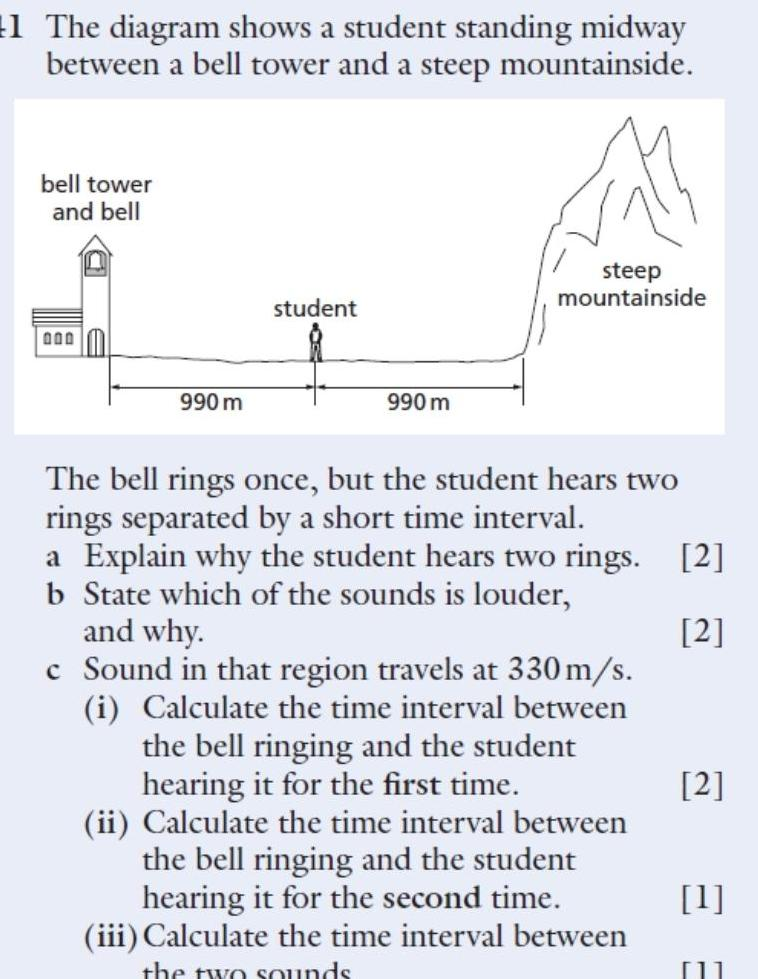Physics
Sound Waves
1 The diagram shows a student standing midway between a bell tower and a steep mountainside bell tower and bell 000 990 m student 990 m steep mountainside The bell rings once but the student hears two rings separated by a short time interval a Explain why the student hears two rings 2 b State which of the sounds is louder and why 2 c Sound in that region travels at 330 m s i Calculate the time interval between the bell ringing and the student hearing it for the first time ii Calculate the time interval between the bell ringing and the student hearing it for the second time iii Calculate the time interval between the two sounds 2 1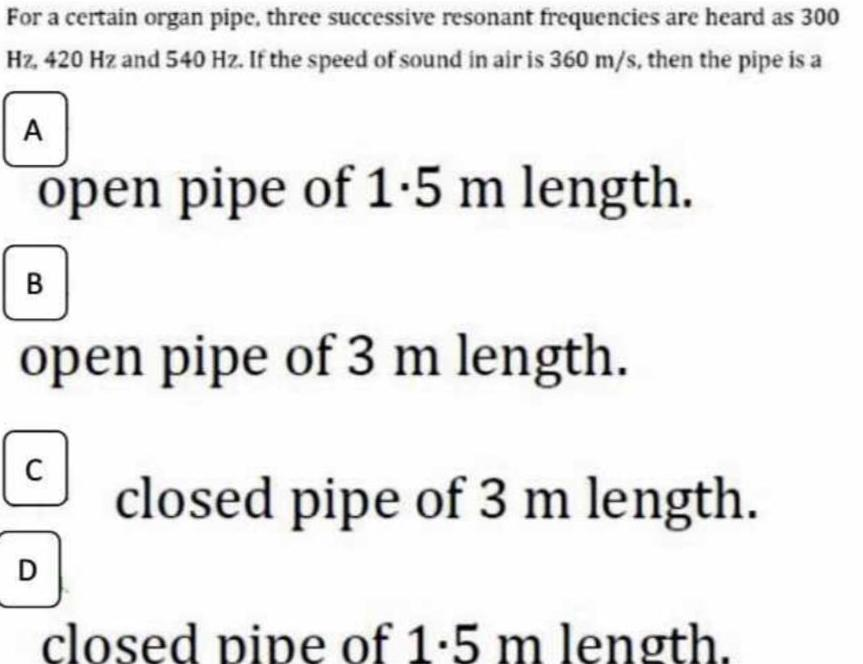Physics
Sound Waves
For a certain organ pipe three successive resonant frequencies are heard as 300 Hz 420 Hz and 540 Hz If the speed of sound in air is 360 m s then the pipe is a A open pipe of 1 5 m length B open pipe of 3 m length C D closed pipe of 3 m length closed pipe of 1 5 m length